# Mathematics - Mathematical Analysis Series Convergence ExamplesHello its a me again drifter1! Today we continue with Mathematical Analysis getting into the promised Series Convergence Examples! I will try covering the most of them, so that you get a basic to advanced understanding of the calculations you need to do. You should check out my previous post about the Convergence Tests before getting into this one though. So, let's get started!

Example 1 (Zero-Limit Test):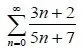The limit to infinity of the sequence (an) inside of the Series is a polynomial limit of equal degrees on numerator and denominator and so equals the quotient of the coefficients of the largest degrees.

This means that lim n->∞ [3n+2 / 5n+7] = 3/5.

Because, this limit is non-zero we know that the Series diverges.

Example 2 (Comparison Test):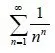For n>=2 we know that n^n >= 2^n something easily provable using Mathematical induction.

Suppose the sequences (an) = 1/n^n and (bn) = 1/2^n.

Because n^n >= 2^n we also know that 0 < an <= bn.

The Series formed of bn terms is a geometric series with p = 2 > 1 and so converges.

The comparison test tells us that if bn converges then an also converges and so the given series converges.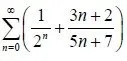The first Series of the sum is the same exact geometric series as in the previous example with p = 2 > 1 and so converges.

The second Series is the same as the first example and because the limit to infinity doesn't give us 0 we know that this Series diverges.

Because, of the addition convergence property we say that: because at least one (one in this example) of the series in the sum diverges, the whole sum also diverges.

This means that the given series diverges.

Example 4 (Limit Comparison):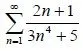The generic term of the series is bn = 2n+1 / 3n^4+5 >=0 for any natural n.

Here the degrees are different and so we have to use another technique called limit comparison.

To do this we will use a temporary helping sequence (an) with a degree equal to the difference of the denominator and numerator of (bn).

Let's take an = 1/n^3 >=0 so that:

an/bn = (1/n^3)/(2n+1 / 3n^4+5) = (3n^4+5) / (2n^4 + n^3)

We get this by multiplying the top-bottom and putting the result on the numerator and the center ones and putting the result in the denominator.

So, the limit is now equal to the quotient of the coefficients of the largest degrees and so 3/2 = k.

Because, S(an) is a p-harmonical series with p = 3 > 1 we know that it converges.

Also, cause k > 0 and not infinity the limit comparison test applies and so S(bn) also converges.

This means that the given Series converges.

Example 5 (Ratio Test):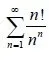an = n! /n^n is a positive sequence for every natural n.

an+1 = (n+1)! / (n+1)^(n+1).

Think about (n+1)! as 1*2*3*....*n*(n+1) = n!*(n+1) is we take (n+1) alone.

Also we will write (n+1)^(n+1) as (n+1)*(n+1)^n.

So, an+1 = (n+1)*n! / (n+1)*(n+1)^(n) = n! / (n+1)^n

This means that an+1 / an = [n! / (n+1)^n] / [ n! /n^n] =

n^n / (n+1)^n = (n/(n+1))^n = 1/ ((n+1)/n)^n.

I did the last thing so that we get the limit that gives us e.

We know that the limit to infinity of (n+1)/n)^n gives us e and so:

lim n->∞ |an/bn| =   lim n->∞ [1/ ((n+1)/n)^n] = 1/e = k < 1.

So, the ratio test applies and the series converges.

Example 6 (n-root Test):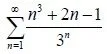an = (n^3 + 2n -1) / 3^n is a positive series for every natural n.

By using the n-root test and so the limit of the (n-root of n) = 1 we end up with:

lim n->∞ [n-root (n^3 + 2n -1) / 3^n] = lim n->∞ [n-root(n^3) / 3] =

lim n->∞ [n-root(n)^3 / 3] = 1^3/3 = 1/3 = k < 1

For the n-root of the polynomial in the numerator we just used the largest degree term.

So, with k<1 the n-root test applies and the given series converges.

Example 7 (Absolute Test):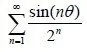This limit first seems difficult, but let's try solving it using the absolute test.

Suppose the sequence an = sin(nθ) / 2^n for any real θ and natural n.

Because, |sin(nθ)| <= 1 for every natural n we say that:

|an| = | sin(nθ) / 2^n | <= 1/2^n.

The second series of bn = 1/2^n terms converges, because its a geometric series with p = 2 >1 (the same example we had earlier in this post).

So, because of the comparison test we say that | sin(nθ) / 2^n | because 1/2^n converges.

Also, cause of the absolute convergence test we say that sin(nθ) / 2^n also converges cause the absolute of that series converges.

Finally we know that the given series converges.

Example 8 (Leibniz Alternating Series Test):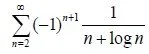The series is alternating with an = 1 / n+logn.

• an is positive for every natural n
• the logarithmic function logn and so the whole denominator is increasing and so the whole sequence is strictly decreasing.
• the limit to infinity gives us 1/∞ = 0 and so the series is a nullsequence

All the conditions of the Leibniz Theorem/Test apply and so the given series converges.

If any of the conditions didn't apply then we would say that the series diverges.

Try solving this one: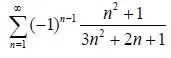This series diverges, cause the nullsequence condition is not met.

Example 9 (Integral Convergence):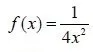Suppose the upper function f.

Does the integral of 1 to +∞ of this function converge?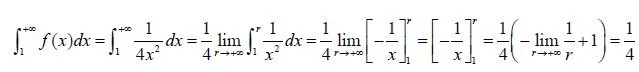By doing the calculations and finding the value we see that the function does indeed converge.

But, can we get the same answer in another faster way?

Well, there is this Integral/Series Convergence Test.

If we suppose a series with term 1/4x^2 then it converges cause its a geometric series with p = 2 > 1.

So, because of the Integral/Series Convergence Test the Integral of the same sequence an = 1/4x^2 in the same range [1, +∞) also converges.

And this is actually it and I hope you enjoyed it and learned something!

Next time we will get into Power Series!

Bye!

H2
H3
H4
3 columns
2 columns
1 column